Quantum frequency doubling based on tripartite entanglement with cavities
College of Physics and Electronic Engineering, Shanxi University, Taiyuan 030006, China
Institute of Theoretical Physics, Shanxi University, Taiyuan 030006, China

† Corresponding author. E-mail: zhangsy@sxu.edu.cn

Project supported by the National Natural Science Foundation of China (Grant No. 91430109), the Specialized Research Fund for the Doctoral Program of Higher Education of China (Grant No. 20111401110004), and the Natural Science Foundation of Shanxi Province, China (Grant No. 2014011005-3).

Abstract
Abstract

We analyze the entanglement characteristics of three harmonic modes, which are the output fields from three cavities with an input tripartite entangled state at fundamental frequency. The entanglement properties of the input beams can be maintained after their frequencies have been up-converted by the process of second harmonic generation. We have calculated the parametric dependences of the correlation spectrum on the initial squeezing factor, the pump power, the transmission coefficient, and the normalized analysis frequency of cavity. The numerical results provide references to choose proper experimental parameters for designing the experiment. The frequency conversion of the multipartite entangled state can also be applied to a quantum communication network.

1. Introduction

Quantum frequency conversion (QFC) has been proposed to connect telecommunication wavelength light (carrier) with atomic transitions (quantum memories)[1,2] and improvement of optical detection, by using the nonlinear effect in nonlinear media χ(2) and χ(3). It can be used to change the frequency of a quantum state to a desired one.[4,5] It is proved that the properties of quantum states of light can be maintained during QFC, including non-classical correlation, entanglement, non-classical photon statistics, photon coherence,[12,13] and orbital angular momentum. Recently, the successful experiments of spectral compression of single photons, infrared or mid-infrared up-conversion imaging,[16,17] and quantum up-conversion of squeezed states[18,19] has aroused interest in exploring more applications of QFC. A direct quantum interface between photonic qubits of widely different wavelengths was demonstrated in Ref. . In their work, the energy–time entanglement of a photon at 1310 nm with a photon at 1550 nm was transferred to that of another photon at a wavelength of 710 nm via sum-frequency generation (SFG). It is verified that the entanglement is unaffected, though one of the two entangled photons is transferred by an up-conversion process. As 710-nm wavelength is close to alkaline atomic transition and the transmission wavelengths for photons in optical fibers are 1310 nm and 1550 nm,[20,21] the converted entanglement can be used for quantum networks based on atoms and photons.

On the other hand, it is known that when three continuous variable (CV) single-mode squeezed states input on two beam splitters, a tripartite CV entangled state is yielded. In Ref. , the squeezing direction of mode 1 is Y, the other modes are squeezed in X as shown in Fig. 1, and the three output modes are all genuinely tripartite entangled states. In this paper, we analyze an intracavity scheme with frequency doubling of the tripartite entangled states. The tripartite entangled fields at 1560 nm are transferred to the three-mode entanglement at 780 nm, by the process of second harmonic generation, which is a special process of up-conversion. As 780 nm is the absorption line of Rb atom, the produced entanglement can be used in an atom system.

 Figure Option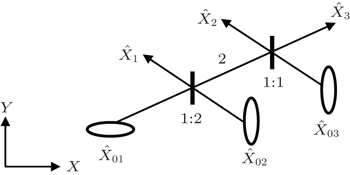Fig. 1. Scheme for producing the tripartite entangled state of modes 1, 2, and 3 with three single squeezed states (one state is squeezed in Y direction, the other two are squeezed in X direction) and two beam splitters (one is 1:2 BS and the other is 1:1).
2. Theoretical model

The scheme of frequency doubling process is depicted in Fig. 2. The tripartite entangled beams from a three-port device called a tritter (Fig. 1),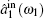,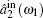, and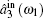, are frequency-degenerated and injected into three one-sided cavities. Each cavity consists of a second-order nonlinear crystal, which provides the interaction of type-I second harmonic generation (SHG). The output fields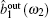,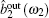, and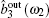are all of frequency ω2 = 2ω1.

 Figure Option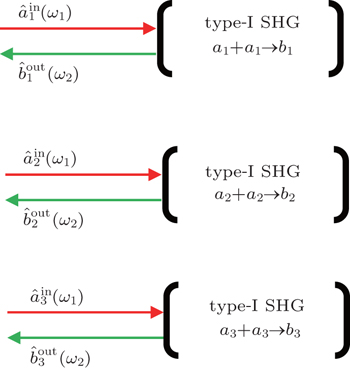Fig. 2. Scheme of physical system for frequency doubling using cavity.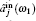are the incoming tripartite entangled fields and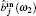are the three outgoing fields. Type-I SHG takes place in each cavity.

The input–output relations of the amplitude quadrature components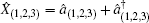and phase quadrature components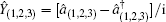on the field modes from the tritter are given in Ref. :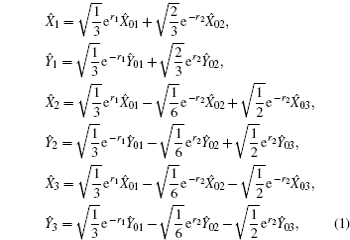where r1 and r2 = r3 are the squeezing factors of the three input lights respectively. The variances of the relative amplitude quadratures and total phase quadratures of three modes are calculated as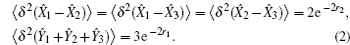It is found that it is easy for the variances to be below the shot noise limit (SNL) and the perfect entanglement can be obtained with infinite squeezing (r1 = r2 → ∞) in single-mode squeezed states.

We will discuss the process of SHG and analyze the preserved tripartite entanglement among the output fields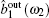,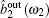,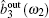in the next section.

3. Frequency doubling process

Under the assumption of perfect phase matching and zero detuning, the Hamiltonian describing the interaction in the cavity can be written as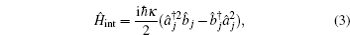where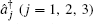is the creation operator of the cavity mode with fundamental frequency and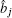is the annihilation operator of the harmonic mode; κ represents the effective nonlinear parameter and is proportional to the nonlinear susceptibility χ(2).

Assuming that nonlinear coefficient κ and loss coefficient γ are the same for all cavities, the quantum Langevin equations of motion for the cavity modes can be expressed as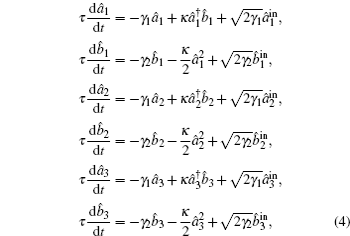where γ1 and γ2 represent the total loss parameters for the fundamental mode and the harmonic mode respectively and are related to the amplitude reflection coefficients rj and amplitude transmission coefficients tj by rj = 1 − γj,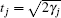; τ denotes the cavity round trip time and is assumed to be the same for all fields;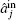and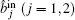denote the operators of the input light fields.

In the symmetric case, we set the steady-state amplitudes of the fundamental modes α1 = α2 = α3 = α and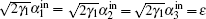. Following the semiclassical method, the threshold to the beginning of the self-pulsing is given by ɛth and the steady-state solutions below the threshold are found to be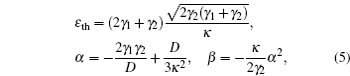where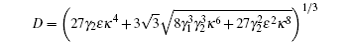and β = β1 = β2 = β3 denote the steady-state amplitudes of the harmonic modes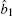,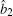, and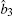.

The linearized equations of motion for quadrature-phase amplitude may be written in a matrix form by setting the following relations j = αj + δ âj,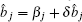, and = δ â + δ â, Ŷ = (δ âδ â)/i,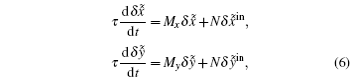with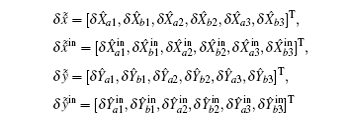and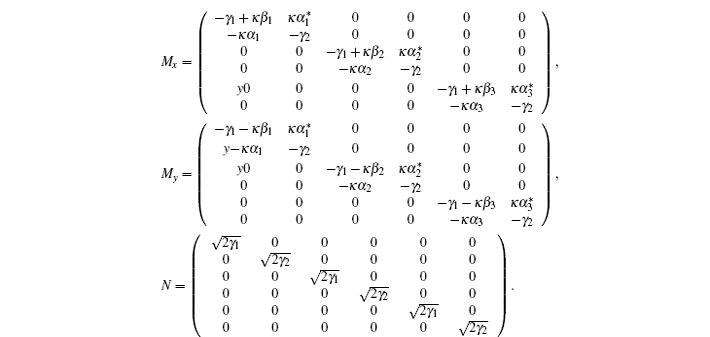According to the boundary condition of the coupling mirrors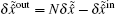,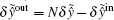, we can obtain the fluctuation of output fields at the analysis frequency ω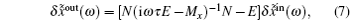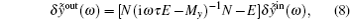where E is the 6 × 6 identity matrix.

4. Entanglement characteristics

According to Eq. (1), the input fluctuation of 1, 2, and 3 can be expressed as: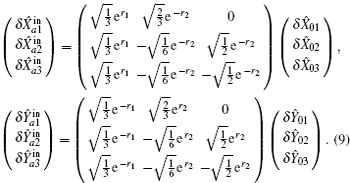By substituting Eq. (9) into Eqs. (7) and (8), we can obtain the correlation spectra to verify the entanglement among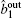,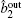, and. Based on the sufficient inseparability criteria for CV tripartite entanglement proposed by van Loock and Furusawa, the following three inequalities should be satisfied at the same time: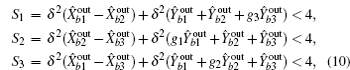where g1, g2, and g3 are scaling factors.

Because the variances of the relative amplitude quadratures and total phase quadratures of the input tripartite entanglement are identical and we have assumed the parameters of the three cavities to be the same, the correlation spectra S1 = S2 = S3 = S. The dependences of S on the normalized analysis frequency Ω = ω τ/γ1 under different squeezed factor are shown in Fig. 3. Obviously, the maximum correlation (S is minimum) is obtained at low frequency. For the low frequency, if the initial squeezing factor r2 = r1 = r becomes larger, the preserved correlation among b1, b2, and b3 is better. But for the high frequency, the smaller the squeezing factor, the better the preserved correlation is, and we have a wider frequency range to measure the entanglement corresponding to smaller r. Figure 4 shows the correlation fluctuation spectra S versus pump parameter σ = ɛ/ɛth for different values of squeezing factor r. The tripartite entanglement is retained at low pump power. It is obvious that the better correlation is obtained with larger r. But for a given r, we have an optimal pump parameter (σ around 0.12) where S reaches a minimum. In addition, the optimal cavity parameters should be chosen to meet the smallest S for preserving the quantum correlation as shown in Fig. 5. If the amplitude transmission coefficient of harmonic mode is twice that of fundamental mode, the maximum entanglement is maintained.

 Figure Option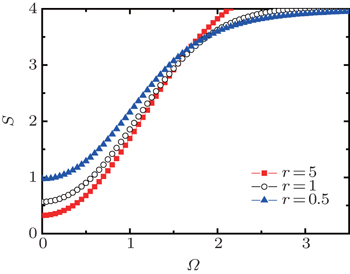Fig. 3. Correlation fluctuation spectra S versus the normalized analysis frequency Ω, pump parameter σ = 0.1, transmission of the output coupler mirror γ2/γ1 = 2. The squares, circles, and triangles are for squeezing factor r = 5, 1, 0.5 respectively.
 Figure Option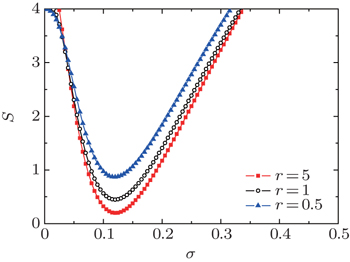Fig. 4. Correlation fluctuation spectra S at Ω = 0 versus pump parameter σ for different values of squeezing factor r, the transmission of the output coupler mirror γ2/γ1 = 2. The squares, circles, and triangles are for squeezing factor r = 5, 1, 0.5 respectively.
 Figure Option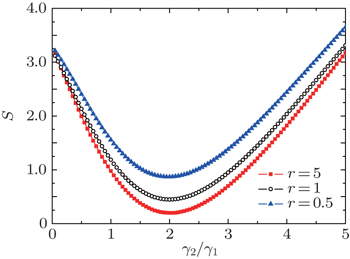Fig. 5. Correlation fluctuation spectra S at Ω = 0 versus transmission of the output coupler mirror γ2/γ1 for different values of squeezing factor (r = 5, 1, 0.5), the pump parameter σ = 0.12. The squares, circles, and triangles are for squeezing factor r = 5, 1, 0.5 respectively.
5. Conclusions

We propose a scheme to preserve the tripartite entanglement based on the process of SHG in three cavities, and it can be realized when the cavity operates far below the threshold. The dependences of the correlation spectrum on the parameters of cavity, the analysis frequency, and the initial squeezing are discussed. The entangled state conversion between different frequencies can be used to connect the atom with light in a quantum communication network, and the experimental scheme can be realized with the matured entanglement technology.

Reference
 1 Radnaev A GDudin Y OZhao RJen H HJenkins S DKuzmich AKennedy T A B 2010 Nat. Phys. 6 894 2 Han YWu C WWu WChen P XLi C Z 2009 Chin. Phys. B 18 3215 3 Vandevender AKwiat P 2004 J. Mod. Opt. 51 1433 4 Kumar P 1990 Opt. Lett. 15 1476 5 Wang LLuo M XSun J XSun Y HChen YWei X GKang Z HWang H HGao J Y 2015 Chin. Phys. B 24 064205 6 Huang JKumar P 1992 Phys. Rev. Lett. 68 2153 7 Tanzilli STittel WHalder MAlibart OBaldi PGisin NZbinden H 2005 Nature 437 116 8 Rakher M TMa LSlattery OTang XSrinivasan K 2010 Nat. Photon. 4 786 9 McGuinness H JRaymer M GMcKinstrie C JRadic S 2010 Phys. Rev. Lett. 105 093604 10 Bai Y FZhai S QGao J RZhang J X 2011 Chin. Phys. B 20 034207 11 Bai Y FZhai S QGao J RZhang J X 2011 Chin. Phys. B 20 084207 12 Zaske SLenhard AKe?ler C AKettler JHepp CArend CAlbrecht RSchulz W MJetter MMichler PBecher C 2012 Phys. Rev. Lett. 109 147404 13 Ates SAgha IGulinatti ARech IRakher M TBadolato ASrinivasan K 2012 Phys. Rev. Lett. 109 147405 14 Tang R KLi X JWu W JPan H FZeng H PWu E 2015 Opt. Express 23 9796 15 Lavoie JDonohue J MWright L GFedrizzi AResch K J 2013 Nat. Photon. 7 363 16 Zhou QHuang KPan H FWu EZeng H P 2013 Appl. Phys. Lett. 102 241110 17 Kehlet L MTidemand-Lichtenberg PDam J SPedersen C 2015 Opt. Lett. 40 938 18 Vollmer C EBaune CSamblowski AEberle THändchen VFiurášek JSchnabel R 2014 Phys. Rev. Lett. 112 073602 19 Baune CGniesmer JSchönbeck AVollmer C EFiurášek JSchnabel R 2015 Opt. Express 23 16035 20 Li X YYang LMa X XCui LOu Z YYu D Y 2009 Phys. Rev. A 79 033817 21 Ding XSheng QChen NYu X YWang RZhang HWen W QWang PYao J Q 2009 Chin. Phys. B 18 4314 22 Lloyd SShahriar M SShapiro J HHemmer P R 2001 Phys. Rev. Lett. 87 167903 23 Loock P VBraunstein S L 2000 Phys. Rev. Lett. 84 3482 24 Braunstein S L 1998 Nature 394 47 25 Collett M JGardiner C W 1984 Phys. Rev. A 30 1386 26 Loock P VFurusawa A 2003 Phys. Rev. A 67 052315